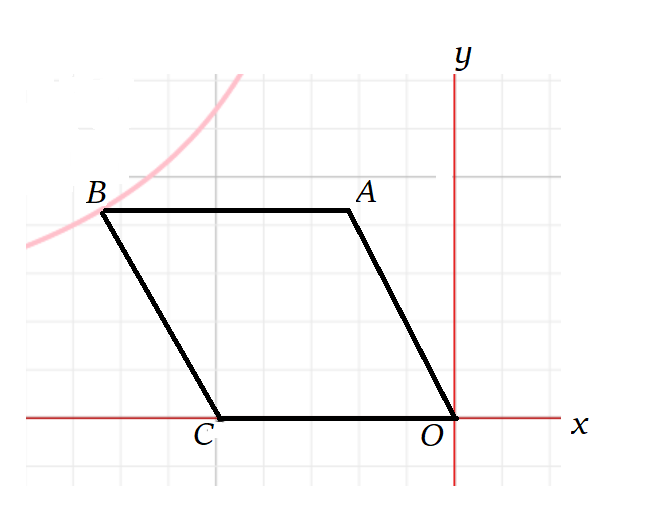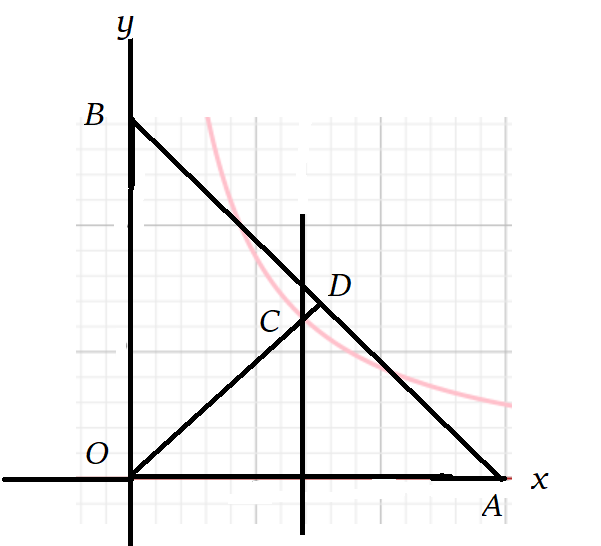# 基本说明

## 反比例函数的图像

### 性质

• 反比例函数的图像是双曲线
• 当 $k>0$ 时，两条分支分别位于第一、三象限内，$y$ 随着 $x$ 的增大二减小；$k<0$ 时，两条分支分别位于第二、四象限内，$y$ 随 $x$ 的增大而增大。
• 两个分支无线逼近于 $x,y$ 轴，但是永远不会相交。

### 画法

#### 列表

$x$$y \cdots$$\cdots$
$-1$$-2 0无意义 1$$2$
$2$$1 3$$\dfrac{2}{3}$
$\cdots$$\cdots$

# 综合运用

## 练习

1. 如图，$O$ 是坐标原点，菱形 $OABC$ 的顶点 $A$ 的坐标为 $(-3,4)$，顶点 $C$ 在 $x$ 轴的负半轴上，函数 $y=\dfrac{k}{x}(x<0)$ 的图像经过顶点 $B$，则 $k$ 的值为___1. 如图，在平面直角坐标系 $xOy$ 中，点 $A,B$ 分别在 $x,y$ 轴上，$\dfrac{OA}{OB}=\dfrac{3}{4}$，$\angle AOB$ 的平分线与 $OA$ 的垂直平分线交于点 $C$，与 $AB$ 交于点 $D$，反比例函数 $y=\dfrac{k}{x}$ 的图像经过点 $C$，当以 $CD$ 为边的正方形的面积是 $\dfrac{2}{7}$ 时，$k$ 的值为___1. 在平面直角坐标系的第一象限内，边长为 $1$ 的正方形 $ABCD$ 的边均平行于坐标轴，点 $A$ 的坐标为 $(a,a)$。若曲线 $y=\dfrac{3}{x}(x>0)$ 与此正方形的边有交点，则 $a$ 的取值范围时是__
2. 点 $(a-1,y_1),(a+1,y_2)$ 在反比例函数 $y=\dfrac{k}{x}(k>0)$ 的图像上，若 $y_1<y_2$，则 $a$ 的取值范围是_____
3. 若 $a,b \in \mathbb{Q}$，$a,b≥0$，则 $a+b≥2\sqrt{ab}$，当且仅当 $a=b$ 时， “$=$”成立。
• 请证明这个结论；
• 已知 $x>0$，求函数 $y=2x+\dfrac{2}{x}$ 的最小值；
• 汽车的经济时速是指汽车最省油的行驶速度，某种跑车在每小时 $70 \text{ km} \text{~} 110 \text{ km}$ 之间行驶的时候，每千米耗油 $(\dfrac{1}{18}+\dfrac{450}{x^2})\text{L}$。若该汽车以每小时 $x \text{ km}$ 的速度匀速行驶，$1 \text{ h}$ 的耗油量为 $y \text{ L}$；
• 求 $y$ 关于 $x$ 的函数关系式；
• 利用上述材料，求这个汽车的经济时速，并求出当跑车以经济时速形式的时候，每 $100 \text{ km}$ 的耗油量（保留一位小数）。